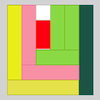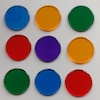# Resources tagged with: Factors and multiples

Filter by: Content type:
Age range:
Challenge level:

### There are 156 results

Broad Topics > Properties of Numbers > Factors and multiples### Sweets in a Box

##### Age 7 to 11Challenge Level

How many different shaped boxes can you design for 36 sweets in one layer? Can you arrange the sweets so that no sweets of the same colour are next to each other in any direction?### It Figures

##### Age 7 to 11Challenge Level

Suppose we allow ourselves to use three numbers less than 10 and multiply them together. How many different products can you find? How do you know you've got them all?### Pebbles

##### Age 7 to 11Challenge Level

Place four pebbles on the sand in the form of a square. Keep adding as few pebbles as necessary to double the area. How many extra pebbles are added each time?### Sets of Four Numbers

##### Age 7 to 11Challenge Level

There are ten children in Becky's group. Can you find a set of numbers for each of them? Are there any other sets?### Ip Dip

##### Age 5 to 11Challenge Level

"Ip dip sky blue! Who's 'it'? It's you!" Where would you position yourself so that you are 'it' if there are two players? Three players ...?### Gran, How Old Are You?

##### Age 7 to 11Challenge Level

When Charlie asked his grandmother how old she is, he didn't get a straightforward reply! Can you work out how old she is?### Multiplication Squares

##### Age 7 to 11Challenge Level

Can you work out the arrangement of the digits in the square so that the given products are correct? The numbers 1 - 9 may be used once and once only.### Sets of Numbers

##### Age 7 to 11Challenge Level

How many different sets of numbers with at least four members can you find in the numbers in this box?### Multiply Multiples 1

##### Age 7 to 11Challenge Level

Can you complete this calculation by filling in the missing numbers? In how many different ways can you do it?### Multiply Multiples 2

##### Age 7 to 11Challenge Level

Can you work out some different ways to balance this equation?### Abundant Numbers

##### Age 7 to 11Challenge Level

48 is called an abundant number because it is less than the sum of its factors (without itself). Can you find some more abundant numbers?### Curious Number

##### Age 7 to 11Challenge Level

Can you order the digits from 1-3 to make a number which is divisible by 3 so when the last digit is removed it becomes a 2-figure number divisible by 2, and so on?### Multiply Multiples 3

##### Age 7 to 11Challenge Level

Have a go at balancing this equation. Can you find different ways of doing it?### Times Tables Shifts

##### Age 7 to 11Challenge Level

In this activity, the computer chooses a times table and shifts it. Can you work out the table and the shift each time?### Seven Flipped

##### Age 7 to 11Challenge Level

Investigate the smallest number of moves it takes to turn these mats upside-down if you can only turn exactly three at a time.### Rearranged Rectangle

##### Age 7 to 11Challenge Level

How many different rectangles can you make using this set of rods?### Tiling

##### Age 7 to 11Challenge Level

An investigation that gives you the opportunity to make and justify predictions.### A Square Deal

##### Age 7 to 11Challenge Level

Complete the magic square using the numbers 1 to 25 once each. Each row, column and diagonal adds up to 65.### The Moons of Vuvv

##### Age 7 to 11Challenge Level

The planet of Vuvv has seven moons. Can you work out how long it is between each super-eclipse?### Mystery Matrix

##### Age 7 to 11Challenge Level

Can you fill in this table square? The numbers 2 -12 were used to generate it with just one number used twice.### Neighbours

##### Age 7 to 11Challenge Level

In a square in which the houses are evenly spaced, numbers 3 and 10 are opposite each other. What is the smallest and what is the largest possible number of houses in the square?### Round and Round the Circle

##### Age 7 to 11Challenge Level

What happens if you join every second point on this circle? How about every third point? Try with different steps and see if you can predict what will happen.### Being Resilient - Primary Number

##### Age 5 to 11Challenge Level

Number problems at primary level that may require resilience.### Fitted

##### Age 7 to 11Challenge Level

Nine squares with side lengths 1, 4, 7, 8, 9, 10, 14, 15, and 18 cm can be fitted together to form a rectangle. What are the dimensions of the rectangle?### Surprising Split

##### Age 7 to 11Challenge Level

Does this 'trick' for calculating multiples of 11 always work? Why or why not?### Two Primes Make One Square

##### Age 7 to 11Challenge Level

Can you make square numbers by adding two prime numbers together?### A Mixed-up Clock

##### Age 7 to 11Challenge Level

There is a clock-face where the numbers have become all mixed up. Can you find out where all the numbers have got to from these ten statements?### Fractions in a Box

##### Age 7 to 11Challenge Level

The discs for this game are kept in a flat square box with a square hole for each. Use the information to find out how many discs of each colour there are in the box.### Zios and Zepts

##### Age 7 to 11Challenge Level

On the planet Vuv there are two sorts of creatures. The Zios have 3 legs and the Zepts have 7 legs. The great planetary explorer Nico counted 52 legs. How many Zios and how many Zepts were there?### Number Tracks

##### Age 7 to 11Challenge Level

Benâ€™s class were cutting up number tracks. First they cut them into twos and added up the numbers on each piece. What patterns could they see?### Multiples Grid

##### Age 7 to 11Challenge Level

What do the numbers shaded in blue on this hundred square have in common? What do you notice about the pink numbers? How about the shaded numbers in the other squares?### Crossings

##### Age 7 to 11Challenge Level

In this problem we are looking at sets of parallel sticks that cross each other. What is the least number of crossings you can make? And the greatest?### Music to My Ears

##### Age 7 to 11Challenge Level

Can you predict when you'll be clapping and when you'll be clicking if you start this rhythm? How about when a friend begins a new rhythm at the same time?### Summing Consecutive Numbers

##### Age 11 to 14Challenge Level

15 = 7 + 8 and 10 = 1 + 2 + 3 + 4. Can you say which numbers can be expressed as the sum of two or more consecutive integers?### Number Detective

##### Age 5 to 11Challenge Level

Follow the clues to find the mystery number.### Diagonal Product Sudoku

##### Age 11 to 16Challenge Level

Given the products of diagonally opposite cells - can you complete this Sudoku?### Gabriel's Problem

##### Age 11 to 14Challenge Level

Gabriel multiplied together some numbers and then erased them. Can you figure out where each number was?### Repeaters

##### Age 11 to 14Challenge Level

Choose any 3 digits and make a 6 digit number by repeating the 3 digits in the same order (e.g. 594594). Explain why whatever digits you choose the number will always be divisible by 7, 11 and 13.### Scoring with Dice

##### Age 7 to 11Challenge Level

I throw three dice and get 5, 3 and 2. Add the scores on the three dice. What do you get? Now multiply the scores. What do you notice?### Factors and Multiples Game

##### Age 7 to 16Challenge Level

A game in which players take it in turns to choose a number. Can you block your opponent?### Always, Sometimes or Never? Number

##### Age 7 to 11Challenge Level

Are these statements always true, sometimes true or never true?### Have You Got It?

##### Age 11 to 14Challenge Level

Can you explain the strategy for winning this game with any target?### Colour Wheels

##### Age 7 to 11Challenge Level

Imagine a wheel with different markings painted on it at regular intervals. Can you predict the colour of the 18th mark? The 100th mark?### Divide it Out

##### Age 7 to 11Challenge Level

What is the lowest number which always leaves a remainder of 1 when divided by each of the numbers from 2 to 10?### Making Pathways

##### Age 7 to 11Challenge Level

Can you find different ways of creating paths using these paving slabs?### Star Product Sudoku

##### Age 11 to 16Challenge Level

The puzzle can be solved by finding the values of the unknown digits (all indicated by asterisks) in the squares of the $9\times9$ grid.### Ducking and Dividing

##### Age 7 to 11Challenge Level

Your vessel, the Starship Diophantus, has become damaged in deep space. Can you use your knowledge of times tables and some lightning reflexes to survive?### Red Balloons, Blue Balloons

##### Age 7 to 11Challenge Level

Katie and Will have some balloons. Will's balloon burst at exactly the same size as Katie's at the beginning of a puff. How many puffs had Will done before his balloon burst?### Three Dice

##### Age 7 to 11Challenge Level

Investigate the sum of the numbers on the top and bottom faces of a line of three dice. What do you notice?### Dicey Array

##### Age 5 to 11Challenge Level

Watch the video of this game being played. Can you work out the rules? Which dice totals are good to get, and why?